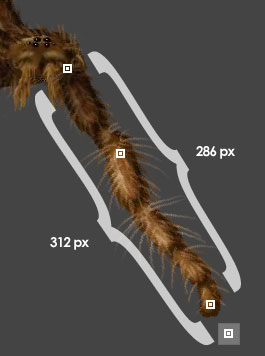Inverse Kinematics Tutorial

February 17, 2004
by Christoffer Enedahl

Prolog

This is my first tutorial. I hope it will be worth your time. This tutorial will only show you the basics of a 2 limbs solution for inverse kinematics (IK). It will not handle angle restrictions, dampening, or any other such features. It will not describe vector math, but you will find a trigonometry library in the source. Basically it will give you the math and source code for calculating the angles of a triangle.

Demo can be viewed here (opens in a new window)

Basics

As you can see in Figure 1, the whole thing is about finding out the angles of a triangle with sides of known lengths. The structure of the bones and IK objects can be found in the source, that is not what I will describe here. Almost all angles in the code are radians but I'll describe them in degrees for better understanding.Figure 1

Here is the function that returns the radian angle x of the far side of a triangle with known lengths. The triangle does not need to be a right triangle. The first argument, c, is the length of the side opposite the corner you want to calculate. You can ignore the order of a and b, they're interchangeable.

-- The code is cut from trigmath v0.6 parentscript.
on GetFarAngle (me,c,a,b)
--
-- |\
-- |x\
-- a | \ b
-- |___\
-- c
--
-- x = a*a + b*b - c*c
-- x = x / float(2*a*b)
-- x = me.InvCos(x)
--
-- return x

if a*b = 0 then return 0

return me.InvCos((a*a+b*b-c*c)/float(2*a*b))
end

on InvCos me, X
y = Sqrt(-X * X + 1)
if y = 0 then return 0--y --NAN
return atan(-X / y) + 2 * pAtan1
end

Let's calculate the angles of our triangle from figure 1

put gTrig.GetFarAngle ( 185, 225 ,104 )
-- 0.9502
-- 54.4424

put gTrig.GetFarAngle ( 225, 104 ,185 )
-- 1.7164
-- 98.3425

OK, so we got a 55° angle for the first bone and 98° angle for the second. If we try to assign those values to the bone, it will behave strangely. We need to offset the angle from the goal angle. The goal angle is the angle between the goal and the first bone's starting position. The goal here is pTarget.

a = pTarget.GetLoc ()
b = pBoneChain.GetLoc ()
vGoalVector = a-b
vGoalAngle = gTrig.VectorToAngle (vGoalVector)
vGoalLength = gTrig.Magnitude2d (vGoalVector)

There is one thing to keep in mind with the trigonometry code. A 0° angle is to the right and 90° angle is down. But for our Director sprite, 0° is up. So we will have to compensate for that by -90°. This compensation really belongs in the drawing routine, but now it ain't. In this case the goal angle is about 35° angle (starting from the right 0° angle)

--First bone
-- vGoalAngle - vCorner1Angle + 90
put +35 - 55 + 90
-- 70

That seems to be correct according to figure 1 with 0° angle up. The next bone is simple, use the angle from the first bone and add the second corner angle

--Second bone
-- vBone.GetTheta() + vCorner1Angle
put 70 + 76
--146

So far, so good. In the source, this part will be slightly messed up by the fact that I have the bone gfx upside down. I know it's kinda stupid. So do better than me.

Now, if you've coded your way here, you'll have a working leg. You should now notice that the leg is bent in one direction only. This is easily fixed, I use a pDirection property in the IK object. It can be -1 or 1, use it to flip the corner angle before calculating the final bone angle. You will need to flipH the sprite as well.

--First bone
-- vGoalAngle - vCorner1Angle*pDirection + 90
put +35 - (55*-1 ) + 90
-- 180
--Second bone
-- vBone.GetTheta() + vCorner2Angle
put 180 + (76*-1)
--104

Early Out

First of all, you should check that the leg can reach the target. If not, point the leg towards the target as in figure 2. There is one case when this code breaks. Try moving the target so close to the leg's starting point that it can't reach it, the leg flips. I haven't tried to fix this yet.Figure 2

That's all folks! That covers the basics, so start coding your own spider or some other beast and show me the results. For more on this subject, see Christophe Leske's Undocumented Lingo site.

Archive files of this Director 8.5-compatible movie are available in ZIP and SIT formats.

Programmer and designer at Amfora Training in Gothenburg, Sweden. A wannabe game developer but never actually seems to finish any games. The artist in him shows now and then in the stuff he does. He is about to become a father in may 2004 and he is very excited, quote: "It will be the best program ever made by me". His personal site is www.enedahl.com.